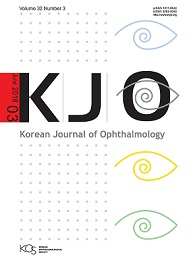상세검색
최근 검색어 전체 삭제
다국어입력
즐겨찾기0KCI등재 학술저널

# Interocular comparison of nerve fiber layer thickness and its relation with optic disc size in normal subjects

• 등재여부 : KCI등재
• 2002.06
• 8 - 12 (5 pages)

We conducted an investigation of the relation between RNFL thickness and optic disc size along with an interocular comparison of optic disc size, RNFL thickness, and RNFL density in healthy subjects. A total of 64 normal eyes from 32 Korean volunteers were enrolled in this study. A GDx Nerve Fiber Analyzer with software version 2.0.09 was used to image all subjects. Optic disc size was measured by π × (horizontal radius) × (vertical radius). The RNFL density of each quadrant was calculated by dividing each quadrant integral by the total integral. Optic disc size was positively correlated with the total RNFL thickness (r = 0.615, p < 0.01). Optic disc size and RNFL density were inversely related in the superior quadrant (r = -0.248, p < 0.05). There was a significant positive correlation between optic disc size and RNFL density in the nasal quadrant (r = 0.439, p < 0.01) and the temporal quadrant to a certain degree. A significant positive correlation was found between the right and left eyes in terms of total RNFL thickness in and that of each quadrant. Interocular RNFL density was positively correlated in both the temporal and nasal quadrants. These findings must be considered when one evaluates and compares RNFL measurements between two eyes as is often the case where both eyes are usually affected in the course of glaucomatous RNFL damage.

Abstract

INTRODUCTION

SUBJECTS AND METHODS

RESULTS

DISCUSSION

REFERENCES

로딩중